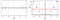# Understanding the kernel trick

## A short explanation of the kernel trick and its relevance to SVMs

Support-vector machines (SVMs) are powerful machine learning models for regression and classification problems. One of the key concepts behind SVMs is the kernel trick, which reduces the complexity of computing nonlinear decision boundaries. Let’s figure out what this means.

Generally speaking, most data are not linearly separable. However, it is possible to transform data to higher-dimensional spaces where they are linearly separable. From there, we can easily compute linear decision boundaries. In the following picture, the green and blue points are not linearly separable. By adding a quadratic feature, however, we can separate them using the dashed red line.Transforming data to a higher-dimensional space to find a linear separation (source)

Let’s frame this in a more rigorous mathematical setting. We are interested in finding a nonlinear classification boundary for the points x by computing a linear classification boundary for the transformed points φ(x). The dual form of the SVM objective is,

When the dimension of φ(x) is high, the inner products on the right are difficult to work out. Kernel functions are functions k such that

The advantage of kernel functions is that they let us compute the values of the inner products in the original, low-dimensional space without having to apply the transformation φ.

So how do we choose a kernel function? In general, if a function k satisfies the conditions of Mercer’s theorem, then there exists a transformation φ such that the above equality holds, meaning we can use k as a kernel. Some common kernels are:

Note that some kernels, such as the sigmoid kernel, do not satisfy Mercer’s conditions but work well in practice.

For a concrete example, consider the simple transformation

Then we have

This corresponds to a polynomial kernel with γ = 1, r = 1, and d = 2.

For more information on kernels and SVMs, I recommend checking out Aurélien Géron’s Hands-On Machine Learning with Scikit-Learn, Keras & TensorFlow.

Machine learning engineer at Holy Grail Inc.

## More from Romain Hardy

Machine learning engineer at Holy Grail Inc.